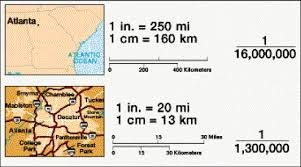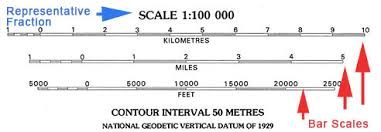# MPA: THE CONCEPT OF SCALE

##### This unit is based on different map scale types

What is Scale?The scale of a map is the ratio between distances on the map and corresponding distances in the real world. In other words, map scale tells the relationship between a distance measured between two points on the map and the actual distance between them on the ground. The scale of a map shows how much how much the given area has been reduced to paper size, and hence how much you would have to enlarge your map to get the actual size of the piece of land shown on the map. For instance, if a map has a scale of 1:50,000, then 1 cm on the map equals 50,000 cm or 0.5 km on the Earth’s surface.

The Map Scale tells the user how the map relates to the real world features it represents. To show a portion of the Earth’s surface on a map, the scale must be sufficiently adjusted to cover the objective. The extent of reduction is expressed as a ratio. The unit on the left indicates distance on the map and the number on the right indicates distance on the ground.

Maps are made at different scales for different purposes. The scale controls not only how features are shown, but what features are shown on a map. For instance, a 1:2,500 map will show individual houses and lamp posts while a 1:100,000, which is a much smaller scale will not show such features.

Types of Map Scale

A map scale can be expressed in three different ways namely representative fraction (RF), statement, and linear. Each of them is discussed below.indicates how many units on the earth’s surface are equal to one unit on the map. It can be expressed as 1/100,000 or 1:100,000. In this example, one centimeter on the map equals 100,000 centimeters (1 kilometer) on the earth. It also means that one inch on the map is equal to 100,000 inches on the land. Other common RFs include 1:63,360 (1 inch to 1 mile) and 1:1,000,000 (1 cm to 10 km). The numerator of a Representative Fraction is always 1. More so, it should be noted that in RF the number on the left hand side (i.e.is the distance or length on map while the figure on the right hand (e.g. 50,000, which is also the denominator) is the corresponding or equivalent length or distance on ground. So the RF 1:10,000 means 1 centimeter on the map represents 10,000 centimeters on the ground (or 1in on the map represents 10,000 inches on the ground).

• A word statement gives a written description of scale, such as “One centimeter equals one kilometer” or “One centimeter equals ten kilometers.” Here, the first map would show much more detail than the second because one centimeter on the first map covers a much smaller area than on the second map.

It should be noted that the two methods mentioned above for indicating scale would be ineffective if the map is reproduced by a method such as photocopying and the size of the map is modified. If this occurs, and one attempts to measure one centimeter on the modified map, it will not be the same as one centimeter on the original map. This can, however, be taken care of by using a linear or graphic scale.

• Linear scale (also known as graphic scale or bar scale – Figure 0) would be able to solve this problem as it is simply a line marked with distance on the ground which the map user can use along with a ruler to determine scale on the map. As long as the size of the graphic scale is changed along with the map, it will be accurate.As shown in Figure , a linear scale is often made up of two component parts namely the primary subdivisions and the secondary subdivisions. The ‘primaries’ are on the right hand side of the zero while the ‘secondaries’ are on the left hand side.

While the primaries are subdivided into kilometers (or miles), the secondaries are subdivided into smaller units such as metres (or furlongs).

• Sizes of Scale

There are three broad categories into which map scales can be grouped. These are small scale, medium scale, and large scale. As a general rule, the higher the denominator the smaller the scale and vice versa.

• Small-Scale maps have scales of about 1:1,000,000 and smaller such as 1:2,000,000; 1:6,000,000; 1:30,000,000 and are used for maps of wide areas. Such maps are used when much detail is not required.

• Medium-Scale maps have scales of 1:50,000; 1: 75,000; 1:100,000 to 1: 1,000,000 and are used for maps of medium sized areas.
• Large-Scale maps have scales larger than 1:50,000 e.g. 1:1000; 1:2,500; 1:5000; 1:10,000 and are used when we want to represent higher levels of detail.

It should be noted that a large scale map shows small features and great detail. On the other hand, a small scale map shows only large features.

Simply put, a ‘large-scale’ map gives a larger and more detailed representation of a feature than does a ‘small-scale’ map. In other words, the smaller the scale the greater is the area which can be shown on a map of given size, but on the other hand we are able to show less and less details. A large-scale map covers a small area but shows more details while a small-scale map covers a large geographical area but gives less detail.

Conversion from One Scale Type to Another

In map reading, one can convert from one scale type to another. For instance, we can convert from statement scale to Representative Fraction (R.F.) and vice versa or from linear scale to statement or RF, and so on.

Examples:

Conversion from R.F. to statement scale

Examples:

Convert the following R.F. scales to statement scale:

(a) 1:1000;  (b) 1:20,000; (c) 1:150,000

Solutions:

NB: 100cm =1m; 100,000cm = 1km

• 1:1000

The R.F. means 1cm represents 1000cm.

1000cm is equal to 10 metres, that is 1000 or 0.01km that is 1000

100

100000

Therefore, the statement scale is 1cm represents 10 metres or 0.01km.

1:20,000

The R.F. means 1cm represents 20,000cm. 20,000cm = 1/5 or 0.2km, that is 20,000

100,000

Therefore, the statement scale is 1cm represents 0.2km or 5cm represent 1km.

• 1:150,000

The R.F. means 1cm represents 150,000cm. 150,000 = 1.5km, that is 150,000

100,000

Therefore, the statement scale is 1cm represents 1.5km or 2cm represents 3km.

Conversion from statement scale to R.F.

Examples:

Convert the following statement scales to Representative Fraction:

(a)       1cm to ½ km   (b)  4cm to 1km

Solutions:

• 1cm to ½ km

The statement scale means 1cm represents ½ or 0.5km. Convert to the same unit of measurement.

1km = 100,000cm

½km = 100,000 = 50,000cm 2

Therefore, the R.F. is 1 or 1:50,000 50,000

4cm to 1km

Convert to the same unit of measurement. 1km = 100,000cm

The statement scale means 4cm represent 100,000cm

If 4cm represent 100,000cm,

1cm will represent 100,000 = 25,000cm

 4 Therefore the R.F. is 1 or 1:25,000 25000

Conversion from Linear scale to statement scale

SEE ALL
•YOU